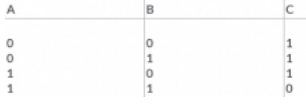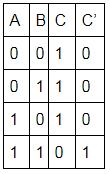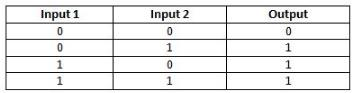Courses

# Test: Logic Gates

## 10 Questions MCQ Test Physics For JEE | Test: Logic Gates

Description
This mock test of Test: Logic Gates for JEE helps you for every JEE entrance exam. This contains 10 Multiple Choice Questions for JEE Test: Logic Gates (mcq) to study with solutions a complete question bank. The solved questions answers in this Test: Logic Gates quiz give you a good mix of easy questions and tough questions. JEE students definitely take this Test: Logic Gates exercise for a better result in the exam. You can find other Test: Logic Gates extra questions, long questions & short questions for JEE on EduRev as well by searching above.
QUESTION: 1

### The binary number system has a base of​

Solution:

Binary number system, in mathematics, positional numeral system employing 2 as the base and so requiring only two different symbols for its digits, 0 and 1, instead of the usual 10 different symbols needed in the decimal system.

QUESTION: 2

### In the binary number 10010, the MSB is​

Solution:

1001=1x23+0x22+0x21+1x20=9
For binary numbers, the digit at the extreme right is referred to as least significant bit (LSB) and the left- most digit is called the most significant bit (MSB). Hence in binary 10010, the most significant bit is 1.

QUESTION: 3

### Which of the following is the universal gate?

Solution:

A universal gate is a gate which can implement any Boolean function without need to use any other gate type. The NAND and NOR gates are universal gates. In practice, this is advantageous since NAND and NOR gates are economical and easier to fabricate and are the basic gates used in all IC digital logic families.

QUESTION: 4

The inputs of a NAND gate are connected together. The resultant circuit is

Solution:

The equation of NAND gate is
Y=Aˉ.Bˉ
When both the terminals are connected together.
A=B=A
Y=Aˉ.Aˉ=Aˉ.Aˉ=Aˉ
Which is nothing but the equation of NOT gate.
Y=Aˉ
Option A is the correct answer.

QUESTION: 5

The NOR gate is OR gate followed by

Solution:

An OR gate followed by a NOT gate in a cascade is called a NOR gate. In other words, the gate which provides a high output signal only when there are low signals on the inputs such type of gate is known as NOR gate.

QUESTION: 6

The truth table given below is for a gate with A and B as inputs and C as output. Which of the gates will obey this truth table?Solution:We can observe from the table that Yˉ=A.B⇒Y=AB
So, the table represents a NAND gate.

QUESTION: 7

Which among the following gates has output = A . B ?​

Solution:

The logic or Boolean expression given for a digital logic AND gate is that for Logical Multiplication which is denoted by a single dot or full stop symbol, ( . ) giving us the Boolean expression of:  A.B = Y.

Then we can define the operation of a digital 2-input logic AND gate as being:
“If both A and B are true, then Y is true”

QUESTION: 8

The output of a 2-input OR gate is zero only when its:

Solution:

The truth table for 2-input OR gate is as shown in the figure. Thus, the output is zero only when both inputs are zero.QUESTION: 9

If A = 1 and B = 0, what is the value of A.A+B?​

Solution:

A=1 and B=0
A.A+B
=A2+B [De-Morgan’s theorem)
=1+0
=1
=A

QUESTION: 10

The binary number 10101 is equivalent to decimal number

Solution:

10101 is the binary number to be converted to a decimal number.
To convert, multiply the numbers individually with 2n where n changes according to place value of the number.
(10101)2= (20× 1) + (0 × 21) + (1×22) + (0×23) + (1×24)
= 1 + 0 + 4 + 0 + 16
= 21